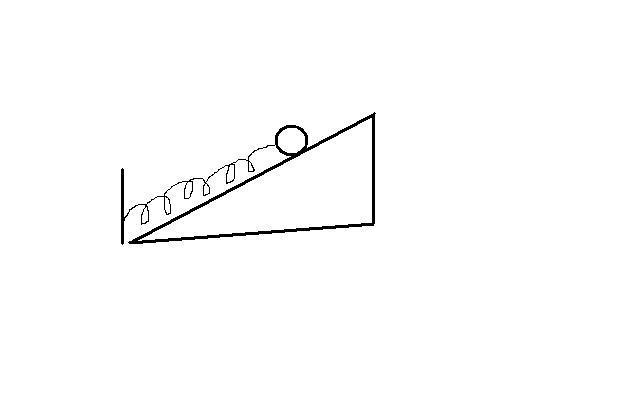A mass (m = 3 kg) is connected to a spring of constant k (k = 50 N/m), where the mass is connected at the top to a spring on a fixed incline. The coefficient of static friction between the mass and the incline is µ(subscript s) = 0.6 and the angle that the incline makes with the horizontal is theta = 20º. The mass is initially held in place so that the spring is at its natural length.

By what maximum amount can the mass be pulled up the incline and released and still have the mass in static equilibrium? Write your final answer as x(subscript s) = ___ centimeters.

Currently I have the equation set up for the force of gravity + force of the spring = force of friction, with 13.05 cm as my disposition. Is this correct and if not then why.

Simon Bridge
Homework Helper
Draw a free body diagram.
Align axis with the slope.
Resolve gravity into components.

You need to sum the forces.
If there was no friction, you could do it right?

The net force on the mass without friction needs to be less than the friction force.
Will gravity and the force from the extended spring act in the same direction?

My work is as follows

(m*g*sinθ)+(Kx)=(μs*m*g*cosθ)

10.055+50x=16.58

50x=6.525

x=13.05 cm

I set the force of gravity and the force of the spring in the same downward direction bc when the spring becomes stretched upwards it wants to return to equilibrium, which is downwards, and the static friction would point in the opposite direction countering those forces, so that it remains in equlibrium.

Simon Bridge
Homework Helper
Well, presumably the spring is hanging from a high point? So you are compressing the spring? I'd just check the wording so you are sure you are displacing the mass in the right direction. Apart from that, you've done what I'd do. Don't forget the fbd in your answer.

Sorry the spring is connected at the bottom of the inclinePeterO
Homework Helper
My work is as follows

(m*g*sinθ)+(Kx)=(μs*m*g*cosθ)

10.055+50x=16.58

50x=6.525

x=13.05 cm

I set the force of gravity and the force of the spring in the same downward direction bc when the spring becomes stretched upwards it wants to return to equilibrium, which is downwards, and the static friction would point in the opposite direction countering those forces, so that it remains in equlibrium.

Your calculations and assigned values look good - that should be the answer.

Thanks so much, my last question is does it make sense that the same disposition of 13.05 cm when it is strectched and remains at static equilibrium would then also apply to when the spring is compressed and remains at static equlibrium. I belive the equation then should look like

(m*g*sinθ)=(Kx)+(μs*m*g*cosθ)

10.055=50X+16.58

-6.525=50x

X= -.1305

13.05 cm

or should friction be pointing downwards with gravity
(m*g*sinθ)+(μs*m*g*cosθ)=Kx
which would then give us 53.27 cm

Simon Bridge
Homework Helper
I concur with PeterO - pat yourself on the back.

 posts passed each other.
check direction of friction

PeterO
Homework Helper
Thanks so much, my last question is does it make sense that the same disposition of 13.05 cm when it is strectched and remains at static equilibrium would then also apply to when the spring is compressed and remains at static equlibrium. I belive the equation then should look like

(m*g*sinθ)=(Kx)+(μs*m*g*cosθ)

10.055=50X+16.58

-6.525=50x

X= -.1305

13.05 cm

or should friction be pointing downwards with gravity
(m*g*sinθ)+(μs*m*g*cosθ)=Kx
which would then give us 53.27 cm

Yes friction is reversed - so the last bit is better [and presumably correctly calculated]